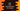# Dart program to find the hash value of a string## How to find the hash value of a string in Dart:

We can find the hash code of a dart string easily. It is an integer value, that is calculated from the code units of the string. It will be always different, only for a same string with same sequence of characters will give the same value. We can calculate the hash value of multiple strings and compare them using == operator.

### Syntax of hashCode:

The syntax of hashCode is as below:

``int get hashCode;``

It is defined in the String class of dart.

### Example of hashCode:

Let’s write a program to find the hashCode of different strings:

``````void main() {
var str_one = "hello";
var str_two = "world";
var str_three = "hello";

print(str_one.hashCode);
print(str_two.hashCode);
print(str_three.hashCode);
}``````

It will print the below output:

``````150804507
278506468
150804507``````

As you can see here, the first and the third print prints the same value because both are same strings.